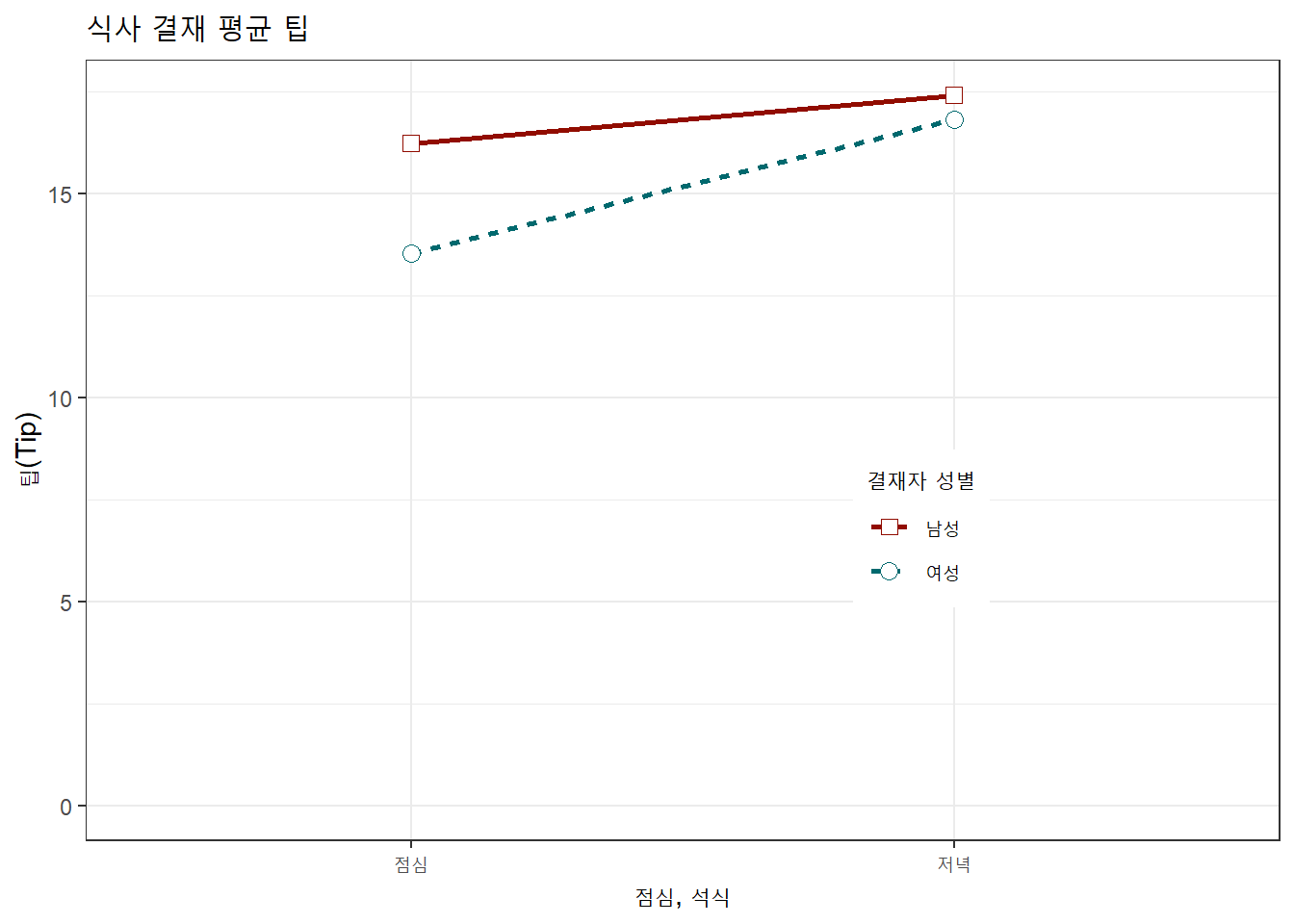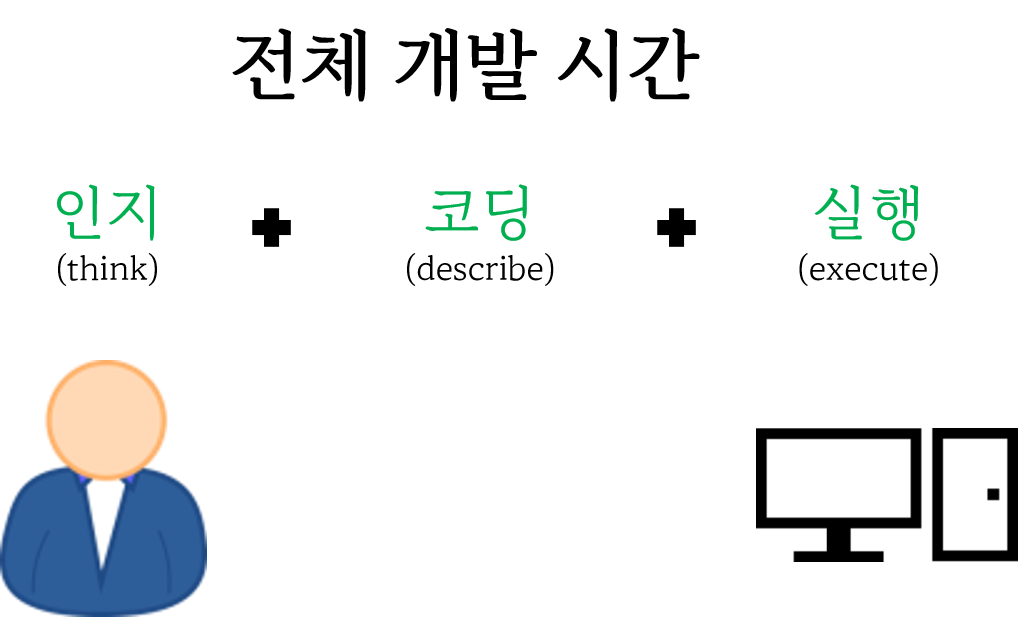데이터 과학 문제

데이터 과학 문제

## 복잡성## 시각화 - 그래프 문법 3

### R 코드

``library(tidyverse)``
``── Attaching packages ─────────────────────────────────────── tidyverse 1.3.1 ──``
``````✔ ggplot2 3.3.6     ✔ purrr   0.3.4
✔ tibble  3.1.7     ✔ dplyr   1.0.9
✔ tidyr   1.2.0     ✔ stringr 1.4.0
✔ readr   2.1.2     ✔ forcats 0.5.1``````
``````── Conflicts ────────────────────────────────────────── tidyverse_conflicts() ──
``````bill_df <- data.frame(
sex = factor(c("여성","여성","남성","남성")),
time = factor(c("점심","저녁","점심","저녁"), levels=c("점심","저녁")),
total_bill = c(13.53, 16.81, 16.24, 17.42)
)``````

### 실행결과

``bill_df``
``````   sex time total_bill
1 여성 점심      13.53
2 여성 저녁      16.81
3 남성 점심      16.24
4 남성 저녁      17.42``````

### R 코드

``````bill_df %>%
ggplot(aes(x=time, y=total_bill, group=sex, shape=sex, colour=sex)) +
geom_line(aes(linetype=sex), size=1) +
geom_point(size=3, fill="white") +
expand_limits(y=0) +
scale_colour_hue(name="결재자 성별", l=30) +
scale_shape_manual(name="결재자 성별", values=c(22,21)) +
scale_linetype_discrete(name="결재자 성별") +
xlab("점심, 석식") + ylab("팁(Tip)") +
ggtitle("식사 결재 평균 팁") +
theme_bw() +
theme(legend.position=c(.7, .4))``````

### 실행결과### R 코드

``````bill_mat <- matrix( bill_df\$total_bill,
nrow = 2,
byrow=TRUE,
dimnames = list(c("여성", "남성"), c("점심", "저녁"))
)

mf_col <- c("#3CC3BD", "#FD8210")
barplot(bill_mat, beside = TRUE, border=NA, col=mf_col)
legend("topleft", row.names(bill_mat), pch=15, col=mf_col)

par(cex=1.2, cex.axis=1.1)
matplot(bill_mat, type="b", lty=1, pch=19, col=mf_col,
cex=1.5, lwd=3, las=1, bty="n", xaxt="n",
xlim=c(0.7, 2.2), ylim=c(12,18), ylab="",
main="식사 결재 평균 팁", yaxt="n")
axis(2, at=axTicks(2), labels=sprintf("\$%s", axTicks(2)),
las=1, cex.axis=0.8, col=NA, line = -0.5)
grid(NA, NULL, lty=3, lwd=1, col="#000000")
abline(v=c(1,2), lty=3, lwd=1, col="#000000")
mtext("점심", side=1, at=1)
mtext("저녁", side=1, at=2)
text(1.5, 17.3, "남성", srt=8, font=3)
text(1.5, 15.1, "여성", srt=33, font=3)``````

### 실행결과## 디자인 - 코드

날짜와 시간별로 총 빈도수와 출발연착 평균 시간을 구한다. 단, 총빈도수가 10 회 미만인 것은 제외하고 출발연착 시간의 결측값을 제거하고 계산한다.

• `flights` 데이터프레임에서
• 출발 연착시간(`dep_delay`) 칼럼에서 결측값이 없는 (`!is.na()`) 행을 필터링 하고
• 날짜별(`date`), 시간별(`hour`) 그룹을 묶어
• 평균 출발 연착시간을 계산하고 총빈도수 총계를 내고
• 총빈도수가 10회 이상인 날짜와 시간을 찾아내시오
``````hourly_delay <- flights %>%
filter(!is.na(dep_delay)) %>%
group_by(date, hour) %>%
summarise(delay = mean(dep_delay), n = n()) %>%
filter(n > 10) ``````
``````hourly_delay <- filter(
summarise(
group_by(
filter(
flights,
!is.na(dep_delay)
),
date, hour
),
delay = mean(dep_delay),
n = n()
),
n > 10
)``````
``````SELECT "date", "hour", "delay", "n"
FROM (
SELECT "date", "hour",
AVG("dep_delay") AS "delay",
COUNT() AS "n"
FROM "flights"
WHERE NOT("dep_delay" IS NULL)
GROUP BY "date", "hour"
) AS "_W1"
WHERE "n" > 10.0``````
``````flights[['date','hour','dep_delay']]
...``````

## 디자인 - 데이터• WHO 결핵 원데이터
• WHO 에서 년도별, 국가별, 연령별, 성별, 진단방법별 결핵 발병사례 조사 통계 데이터
• 진단방법
• `rel` stands for cases of relapse
• `ep` stands for cases of extrapulmonary TB
• `sn` stands for cases of pulmonary TB that could not be diagnosed by a pulmonary smear (smear negative)
• `sp` stands for cases of pulmonary TB that could be diagnosed by a pulmonary smear (smear positive)
• 연령
• 014 = 0 – 14 years old
• 1524 = 15 – 24 years old
• 2534 = 25 – 34 years old
• 3544 = 35 – 44 years old
• 4554 = 45 – 54 years old
• 5564 = 55 – 64 years old
• 65 = 65 or older
• 성별
• males (m)
• females (f)
``````library(tidyverse)
tidyr::who ``````
``````# A tibble: 7,240 × 60
country  iso2  iso3   year new_sp_m014 new_sp_m1524 new_sp_m2534 new_sp_m3544
<chr>    <chr> <chr> <int>       <int>        <int>        <int>        <int>
1 Afghani… AF    AFG    1980          NA           NA           NA           NA
2 Afghani… AF    AFG    1981          NA           NA           NA           NA
3 Afghani… AF    AFG    1982          NA           NA           NA           NA
4 Afghani… AF    AFG    1983          NA           NA           NA           NA
5 Afghani… AF    AFG    1984          NA           NA           NA           NA
6 Afghani… AF    AFG    1985          NA           NA           NA           NA
7 Afghani… AF    AFG    1986          NA           NA           NA           NA
8 Afghani… AF    AFG    1987          NA           NA           NA           NA
9 Afghani… AF    AFG    1988          NA           NA           NA           NA
10 Afghani… AF    AFG    1989          NA           NA           NA           NA
# … with 7,230 more rows, and 52 more variables: new_sp_m4554 <int>,
#   new_sp_m5564 <int>, new_sp_m65 <int>, new_sp_f014 <int>,
#   new_sp_f1524 <int>, new_sp_f2534 <int>, new_sp_f3544 <int>,
#   new_sp_f4554 <int>, new_sp_f5564 <int>, new_sp_f65 <int>,
#   new_sn_m014 <int>, new_sn_m1524 <int>, new_sn_m2534 <int>,
#   new_sn_m3544 <int>, new_sn_m4554 <int>, new_sn_m5564 <int>,
#   new_sn_m65 <int>, new_sn_f014 <int>, new_sn_f1524 <int>, …``````
``````who %>%
pivot_longer(
cols = new_sp_m014:newrel_f65,
names_to = "key",
values_to = "cases",
values_drop_na = TRUE
) %>%
mutate(
key = stringr::str_replace(key, "newrel", "new_rel")
) %>%
separate(key, c("new", "var", "sexage")) %>%
select(-new, -iso2, -iso3) %>%
separate(sexage, c("sex", "age"), sep = 1)``````
``````# A tibble: 76,046 × 6
country      year var   sex   age   cases
<chr>       <int> <chr> <chr> <chr> <int>
1 Afghanistan  1997 sp    m     014       0
2 Afghanistan  1997 sp    m     1524     10
3 Afghanistan  1997 sp    m     2534      6
4 Afghanistan  1997 sp    m     3544      3
5 Afghanistan  1997 sp    m     4554      5
6 Afghanistan  1997 sp    m     5564      2
7 Afghanistan  1997 sp    m     65        0
8 Afghanistan  1997 sp    f     014       5
9 Afghanistan  1997 sp    f     1524     38
10 Afghanistan  1997 sp    f     2534     36
# … with 76,036 more rows``````

## 디자인 - 사람 대 기계## Footnotes

1. Comparing ggplot2 and R Base Graphics By Nathan Yau↩︎